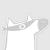0+0+0+0+0+0+We propose a new statistical approach to obtain differential gene expression of non-detects in quantitative real-time PCR (qPCR) experiments through Bayesian hierarchical modeling. We propose to treat non-detects as non-random missing data, model the missing data mechanism, and use this model to impute Ct values or obtain direct estimates of relevant model parameters. A typical laboratory does not have the resources to perform experiments with a large number of replicates; therefore, we propose an approach that does not rely on large sample theory. We aim to demonstrate the possibilities that exist for analyzing qPCR data in the presence of non-random missingness through the use of Bayesian estimation. Bayesian analysis typically allows for smaller data sets to be analyzed without losing power while retaining precision. The heart of Bayesian estimation is that everything that is known about a parameter before observing the data (the prior) is combined with the information from the data itself (the likelihood), resulting in updated knowledge about the parameter (the posterior). In this work we introduce and describe our hierarchical model and chosen prior distributions, assess the model sensitivity to the choice of prior, perform convergence diagnostics for the Markov Chain Monte Carlo, and present the results of a real data application.0+0+0+下载预览0+0+0+0+0+0+Human keypoint detection from a single image is very challenging due to occlusion, blur, illumination and scale variance of person instances. In this paper, we find that context information plays an important role in addressing these issues, and propose a novel method named progressive context refinement (PCR) for human keypoint detection. First, we devise a simple but effective context-aware module (CAM) that can efficiently integrate spatial and channel context information to aid feature learning for locating hard keypoints. Then, we construct the PCR model by stacking several CAMs sequentially with shortcuts and employ multi-task learning to progressively refine the context information and predictions. Besides, to maximize PCR's potential for the aforementioned hard case inference, we propose a hard-negative person detection mining strategy together with a joint-training strategy by exploiting the unlabeled coco dataset and external dataset. Extensive experiments on the COCO keypoint detection benchmark demonstrate the superiority of PCR over representative state-of-the-art (SOTA) methods. Our single model achieves comparable performance with the winner of the 2018 COCO Keypoint Detection Challenge. The final ensemble model sets a new SOTA on this benchmark.0+0+0+下载预览0+0+0+0+0+0+Given a data matrix $\mathbf{A} \in \mathbb{R}^{n \times d}$, principal component projection (PCP) and principal component regression (PCR), i.e. projection and regression restricted to the top-eigenspace of $\mathbf{A}$, are fundamental problems in machine learning, optimization, and numerical analysis. In this paper we provide the first algorithms that solve these problems in nearly linear time for fixed eigenvalue distribution and large n. This improves upon previous methods which have superlinear running times when both the number of top eigenvalues and inverse gap between eigenspaces is large. We achieve our results by applying rational approximations to reduce PCP and PCR to solving asymmetric linear systems which we solve by a variant of SVRG. We corroborate these findings with preliminary empirical experiments.0+0+0+下载预览0+0+0+We study least squares linear regression over $N$ uncorrelated Gaussian features that are selected in order of decreasing variance. When the number of selected features $p$ is at most the sample size $n$, the estimator under consideration coincides with the principal component regression estimator; when $p>n$, the estimator is the least $\ell_2$ norm solution over the selected features. We give an average-case analysis of the out-of-sample prediction error as $p,n,N \to \infty$ with $p/N \to \alpha$ and $n/N \to \beta$, for some constants $\alpha \in [0,1]$ and $\beta \in (0,1)$. In this average-case setting, the prediction error exhibits a "double descent" shape as a function of $p$. We also establish conditions under which the minimum risk is achieved in the interpolating ($p>n$) regime.0+0+0+下载预览0+0+0+0+0+0+0+0+0+0+0+0+
Top